Operational Amplifiers - 1

# Operational Amplifiers - 1 Notes | Study Analog Circuits - Electronics and Communication Engineering (ECE)

## Document Description: Operational Amplifiers - 1 for Electronics and Communication Engineering (ECE) 2022 is part of Function Generator & 555 Timer for Analog Circuits preparation. The notes and questions for Operational Amplifiers - 1 have been prepared according to the Electronics and Communication Engineering (ECE) exam syllabus. Information about Operational Amplifiers - 1 covers topics like Introduction and Operational Amplifiers - 1 Example, for Electronics and Communication Engineering (ECE) 2022 Exam. Find important definitions, questions, notes, meanings, examples, exercises and tests below for Operational Amplifiers - 1.

Introduction of Operational Amplifiers - 1 in English is available as part of our Analog Circuits for Electronics and Communication Engineering (ECE) & Operational Amplifiers - 1 in Hindi for Analog Circuits course. Download more important topics related with Function Generator & 555 Timer, notes, lectures and mock test series for Electronics and Communication Engineering (ECE) Exam by signing up for free. Electronics and Communication Engineering (ECE): Operational Amplifiers - 1 Notes | Study Analog Circuits - Electronics and Communication Engineering (ECE)
 1 Crore+ students have signed up on EduRev. Have you?

Introduction

An operational amplifier (Op-Amp) is an integrated circuit that amplifies the difference between two input voltages and produces a single output. From signal point of view, the Op-Amp has two input terminals and one output terminal as shown in figure below.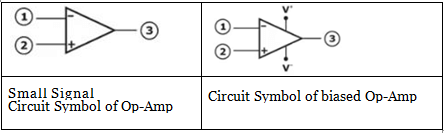Characteristics of Ideal Op-Amp

The ideal Op-Amp senses the difference between two input signals and amplifies this difference to produce an output signal. The output terminal voltage is the voltage at the output terminal measured with respect to ground.
The ideal Op Amp equivalent is shown in figure below: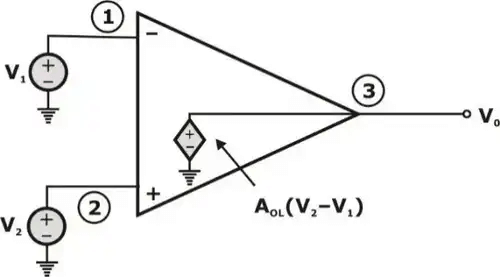Here,
AOL = open loop gain

1. It has infinite input impedance and zero output impedance.
2. The common mode gain is zero (or) equivalently, common mode rejection ratio is infinite.
3. The open loop gain of ideal op-amp is infinite
4. The ideal op-amp has infinite bandwidth and infinite slew-rate.
5. We stated that if the open loop gain (AOL) is very high, then the two input V1 and V2 must be nearly equal. Since, if V2 is at ground potential, voltage V1 must also be approximately zero volts as shown below.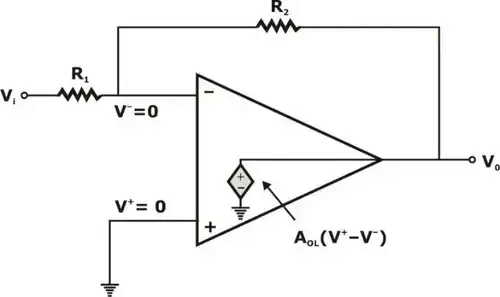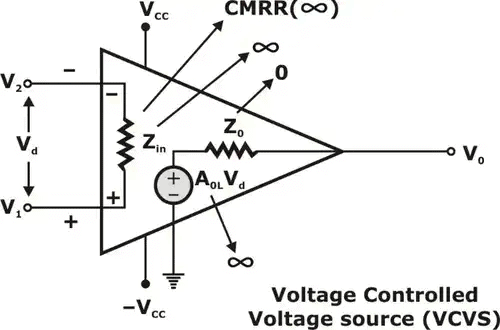Voltage Controlled Voltage Source

Transfer Characteristics of Op Amp

Transfer characteristics equation of Op-Amp: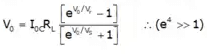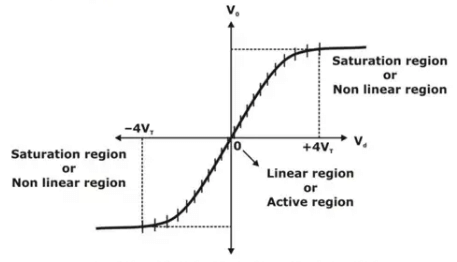Transfer characteristics of Op Amp

Feedback in Op Amp

1. Negative Feedback: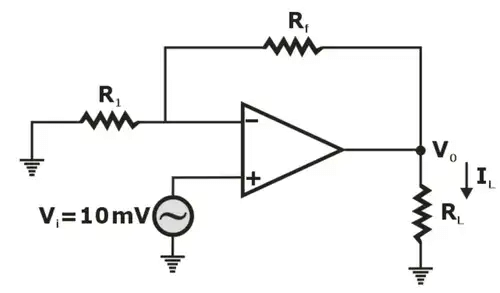Non-Inverting Amplifier
Here, Closed loop gain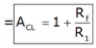Conclusion: When the Op-Amp relates to negative feedback, the voltage gain will reduce.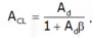2. Positive Feedback: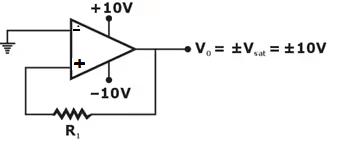Positive Feedback Amplifier

Note: Multivibrator works in open or closed loop of positive feedback.

Virtual-Ground and Comparator

• Virtual ground theory is applicable only in “Negative feedback”. It is not applicable in positive feedback and open loop.
• Comparator theory is applicable for open loop and positive feedback.
Table: Comparison between Negative feedback and Open-loop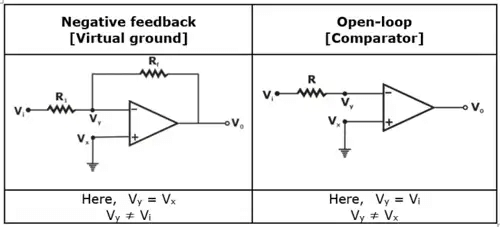Slew-Rate

Slew rate is defined as the maximum rate at which amplifier output can change. It is expressed in Volts per microsecond (V/μs) i.e.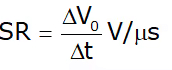Here, ∆V0 = Small change in output voltage in a small interval ∆t.
In terms of input voltage, slew rate can be expressed as: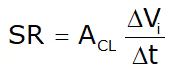Here, ACL = closed loop gain,
∆Vi = Small change in input voltage in a small interval ∆t

Differential and Common Mode operation

1. Differential Inputs
When separate inputs are applied to the op-Amp, the resulting difference signal is the difference between the two inputs
i.e.
Vd = Vi1 – Vi2
Here, Vi1, Vi2 = inputs to the op-Amp.
2. Common Inputs
If there is no difference between the input signals, a common signal element due to the two input signals can be defined as the average of the sum of the two signals.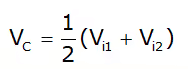Here, Vi1, Vi2 are the inputs to the Op-Amp.
3. Output-Voltage

Since, any signal applied to an Op-Amp is generally have both in phase and out of phase components, the resulting output can be expressed as:
Here,
Vc = Common voltage
Ad = Differential gain of the amplifier
Ac = common mode gain of the amplifier

4. Common Mode Rejection Ratio: (CMRR)
CMRR is defined as the ratio of differential voltage gain to the common mode gain.
In decibels, we may express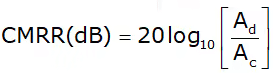Applications of Operational amplifiers

• Inverting Amplifier
• Non-inverting Amplifier
• Differentiator
• Differential Amplifier
• Voltage follower
• Selective inversion circuit
• Current-to-voltage converter
• Active rectifier
• Integrator
• Comparator
• Filters
• Voltage comparator
• Signal Amplifier
1. Inverting-Amplifier
The voltage gain for the inverting Amplifier is given by:
Av = -Rf/R1
Below figure shows the inverting amplifier.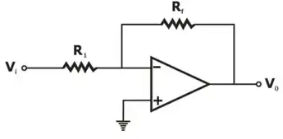2. Non-Inverting Amplifier
The voltage gain for the non-inverting amplifier is given by: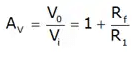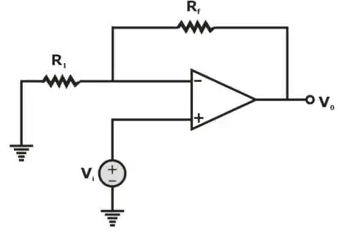Non-Inverting Amplifier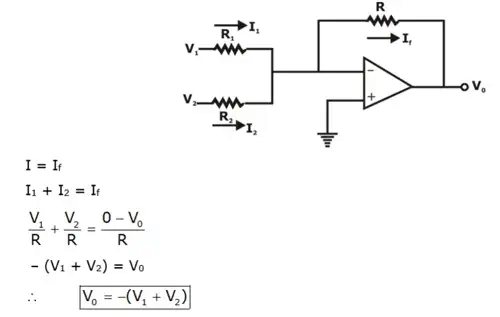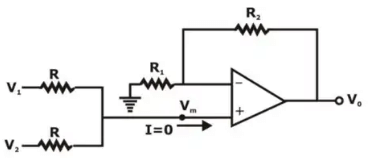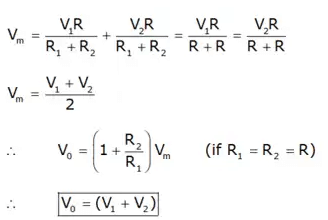4. Voltage Subtractor Circuit
A Voltage Subtractor circuit consist of an inverting amplifier and a summing amplifier. Output of the inverting amplifier is given by: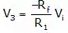So, the output voltage of the summing amplifier is obtained as: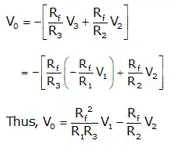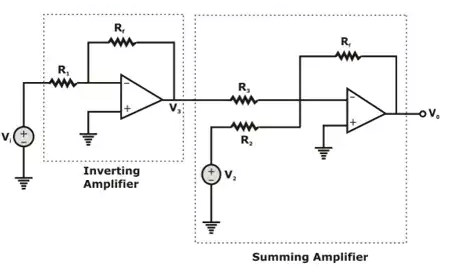Voltage Subtractor Circuit
5. Difference Amplifier
Consider the difference amplifier shown below: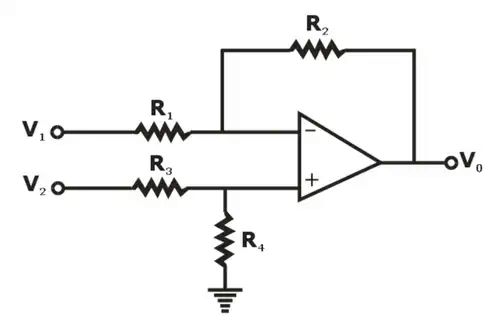Applying Superposition, we consider only one input at a time as shown in below figures.When only V1 is present,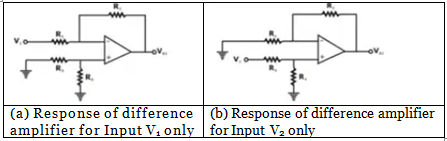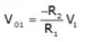When only source V2 Is present, the output Is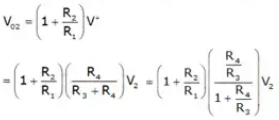Now, on adding V01 and V02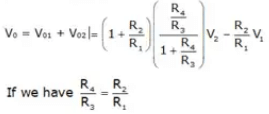Then, the net output voltage = V0 = R2/R1 (V2 - V1)
6. Differentiator Circuit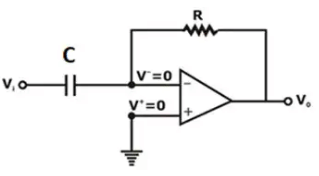Differentiator circuit
Applying KCL at Inverting node, we have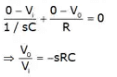It exhibits a zero at origin, so the circuit acts as a differentiator (high pass filter) We can also use the input-output relationship in time domain as: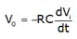7. Integrator Circuit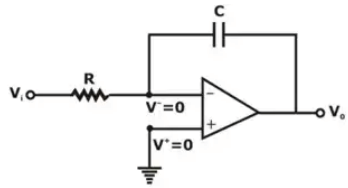Applying KCL at inverting terminal, we have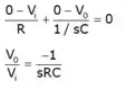Since, the transfer function shows a pole at the origin, the circuit operates as an Integrator (low-pass filter)
Input-output relation in time domain Is —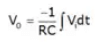The document Operational Amplifiers - 1 Notes | Study Analog Circuits - Electronics and Communication Engineering (ECE) is a part of the Electronics and Communication Engineering (ECE) Course Analog Circuits.
All you need of Electronics and Communication Engineering (ECE) at this link: Electronics and Communication Engineering (ECE)

## Analog Circuits

1 videos|17 docs|43 tests
 Use Code STAYHOME200 and get INR 200 additional OFF

## Analog Circuits

1 videos|17 docs|43 tests

Track your progress, build streaks, highlight & save important lessons and more!

,

,

,

,

,

,

,

,

,

,

,

,

,

,

,

,

,

,

,

,

,

;